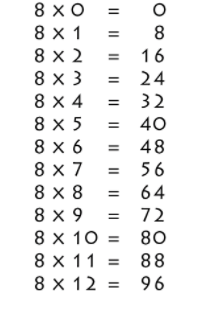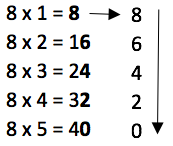# 8 Times Table- Learn Table Of 8 : Multiplication Table Of 8

Safalta expert Published by: Yashaswi More Updated Fri, 08 Sep 2023 05:04 PM IST

## Highlights

Check out how to learn the 8 Times table easily here at Safalta.com

### Free Demo Classes

The 8 times table represents the outcome of multiplying 8 by other whole numbers. Remembering the 8 Table improves memory skills, which is a transferrable skill that will benefit students throughout their educational journey and in life. Students who comprehend the table of eights tend to be more confident in their math abilities. One interesting feature about the number 8 is that when it is flipped, it becomes the symbol of infinity (∞). In this session, you will study the 8 multiplication table with whole numbers up to 20 and several memory tricks.Source: Safalta.com

This lesson will teach you how to memorize an eight-digit multiplication table for whole integers up to twenty, also known as the "8 times table."You may also read 4 Times Table- Learn Table Of 4

For example., Kelly reads 8 pages of a book each day. How many pages will she have read in 9 days? In this, the answer will be 8 x 9 = 72. Join Safalta School Online and prepare for Board Exams under the guidance of our expert faculty. Our online school aims to help students prepare for Board Exams by ensuring that they have conceptual clarity in all the subjects and can score their maximum in the exams.

Also, check,

## 8 times table Chart-## Multiplication Table of 8

• Learning the multiplication table of 8 helps you do mental math. This table comes in handy while solving problems in the real world.
• It saves time while doing long multiplication and division problems.
• The students will be able to recognize the multiplication sequence being followed with the successive multiples of 8.

Go through the 8 times table that is given below for quick calculations.

## 8 Times Table

8 Times Table up to 10

## Tips for 8 Times Table

1. 8 doesn’t have any rules that make its multiplication table of 8 easy to memorize, but there is a pattern for every five multiples of eight. 8, 16, 24, 32, 40, 48, 56, 64, 72, 80.

2. The last digit of these multiples always repeats, which means that students can remember these digits to help them with the 8 times table.

Another way to learn 8 Times Table is-You can notice in the above image that the digits that are in the unit's place occur in a sequence 0, 2, 4, 6, 8 from bottom to top and then recur again. While in the tens place, the numbers are just increasing from top to bottom. We have obtained the first ten multiples of 8. Let us use the same pattern and create the 8 times table up to 20.

## Practice Questions

### (iii) How many eights in 88?

Check

Here we have provided multiplication tables from 2 to 20. Students can learn these math tables from the given below links:

 11 Times Table 3 Times Table 12 Times Table 4 Times Table 13 Times Table 5 Times Table 14 Times Table 6 Times Table 15 Times Table 7 Times Table 16 Times Table 8 Times Table 17 Times Table 9 Times Table 18 Times Table 10 Times Table 19 Times Table 20 Times  Table

The results of multiplying 8 by various whole numbers are displayed in the 8 table. Memory is a transferable talent that will help students throughout their educational careers and lives, and learning the 8 times table will help them get better at it. Students who can understand the table of eights are more confident in their mathematical skills. An intriguing property of the number 8 is that, when turned over, it represents infinity (). You will learn how to memorize an eight-times table in this lesson, which is an eight-by-eight multiplication table containing whole numbers up to twenty. Kelly, for instance, reads 8 pages of a book every day. In nine days, how many pages will she have read? In this, the answer will be 8 x 9 = 72. Join Safalta School Online and prepare for Board Exams under the guidance of our expert faculty. Our online school aims to help students prepare for Board Exams by ensuring that they have conceptual clarity in all the subjects and can score their maximum in the exams.

1. 8 Times Table Chart
2. Multiplication Table of 8
3. 8 Times Table
4. Tips for 8 times table
5. 8 Times table up to 20
6. Practise Questions

## 8 times table Chart-## Multiplication Table of 8

• Learning the multiplication table of 8 helps you do mental math. This table comes in handy while solving problems in the real world.
• It saves time while doing long multiplication and division problems.
• The students will be able to recognize the multiplication sequence being followed with the successive multiples of 8.

Go through the 8 times table that is given below for quick calculations.

## Tips for 8 Times Table

1. 8 doesn’t have any rules that make its multiplication table of 8 easy to memorize, but there is a pattern for every five multiples of eight. 8, 16, 24, 32, 40, 48, 56, 64, 72, 80.

2. The last digit of these multiples always repeats, which means that students can remember these digits to help them with the 8 times table.

Another way to learn 8 Times Table is-You can notice in the above image that the digits that are in the unit's place occur in a sequence of 0, 2, 4, 6, and 8 from bottom to top and then recur again. While in the tens place, the numbers are just increasing from top to bottom. We have obtained the first ten multiples of 8. Let us use the same pattern and create the 8 times table up to 20.

## Practice Questions

1 . Jack is 5 years old. His father is 8 times older than him. How old is the father?

2. Name the number just after:

(i) 5 × 8

(ii) 3 × 8

(iii) 4 × 8

(iv) 10 × 8

(v) 12 × 8

3. A regular octagon has eight equal sides. How many sides do 4 octagons have?
4. An octopus has eight legs. How many legs do 9 octopuses have?
5.  (i) How many eights in 32?

(ii) How many eights in 56?

(iii) How many eights in 88?

1. ### Example 1: Using the table of 8, evaluate 8 times 5 plus 3.

Solution:

This statement is expressed as 8 × 5 + 3

Now, from the 8 times table, we know that 8 times 5 is 40.

Therefore, 8 × 5 + 3 = 40 + 3 = 43

Hence, 8 times 5 plus 3 is 43.

2. ### Example 2: Check using the table of 8 whether 8 times 2 minus 6 is 10.

Solution:

First, we will write 8 times 2 minus 6 mathematically.

Using the 8 times table, we have: 8 times 2 minus 6 = 8 × 2 - 6 = 16 - 6 = 10

Hence, 8 times 2 minus 6 is 10.

3. ### Example 3: Using the 8 times table, find the value of 8 times 3 minus 10 plus 9.

Solution:

First, we will write 8 times 3 minus 10 plus 9 mathematically.

i.e. 8 times 3 minus 10 plus 9 = 8 × 3 - 10 + 9

Now from 8 times table, we have 24 - 10 + 9 = 24 - 1 = 23

Hence, 8 times 3 minus 10 plus 9 is 23.

## Multiplication Tables

 2 Times Table 11 Times Table 3 Times Table 12 Times Table 4 Times Table 13 Times Table 5 Times Table 14 Times Table 6 Times Table 15 Times Table 7 Times Table 16 Times Table 8 Times Table 17 Times Table 9 Times Table 18 Times Table 10 Times Table 19 Times Table 20 Times Table

## What is the multiplication table of 8?

8, 16, 24, 32, 40, 48, 56, 64, 72, 80.

## Does 100 come in the table of 8?

Forward counting by 8's: 0, 8, 16, 24, 32, 40, 48, 56, 64, 72, 80, 88, 96, 104, 112, 120, 128, 136, 144, 152, 160, 168, 176, 184, 192, 200,

## What are the multiples of 8?

The multiples of 8 are 8, 16, 24, 32, 40, 48, 56, 64, 72, 80… and so on. It is a sequence where the difference between each next number and the preceding number, i.e. two consecutive results, is 8.

## What is the importance of learning Table 2 to 20?

For making mathematical section easier, memorising table 2 to 20 is important.

## How can I learn tables easily?

Daily recite the table as mentioned in the article twice and thrice.

## Is 200 in the 8 times table?

Forward counting by 8's: 0, 8, 16, 24, 32, 40, 48, 56, 64, 72, 80, 88, 96, 104, 112, 120, 128, 136, 144, 152, 160, 168, 176, 184, 192, 200,

## Start Learning & Earning

### Trending Courses##### Master Certification in Digital Marketing Programme (Batch-7)

Now at just ₹ 64999 ₹ 12500048% off##### Professional Certification Programme in Digital Marketing!

Now at just ₹ 49999 ₹ 9999950% off##### Advanced Certification in Digital Marketing Online Programme (Batch-18)

Now at just ₹ 24999 ₹ 3599931% off##### Advanced Certification in Digital Marketing CLASSROOM PROGRAMME

Now at just ₹ 44999 ₹ 9999955% off##### Basic Digital Marketing Course (Batch-23): 50 Hours Live+ Recorded Classes!

Now at just ₹ 1499 ₹ 999985% offNow at just ₹ 599 ₹ 159963% off##### Advance Graphic Designing Course (Batch-7) : 150 Hours of Learning

Now at just ₹ 12999 ₹ 2999957% off